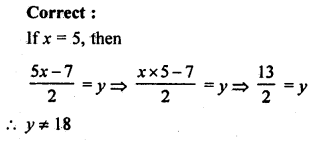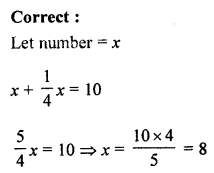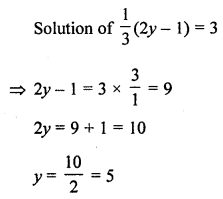## ML Aggarwal Class 7 Solutions for ICSE Maths Chapter 9 Linear Equations and Inequalities Objective Type Questions

Mental Maths

Question 1.
Fill in the blanks:
(i) A linear equation in one variable cannot have more than ………… solution.
(ii) If five times a number is 50, then the number is ……….
(iii) The number 4 is the ………. of the equation 2y – 5 = 3.
(iv) The equation for the statement ‘5 less than thrice a number x is 7’ is ……….
(v) …………. is a solution of the equation 4x + 9 = 5.
(vi) If 3x + 7 = 1, then the value of 5x + 13 is ………..
(vii) In natural numbers, 4x + 5 = -7 has ……….. solution.
(viii) In integers, 3x – 1 = 4 has …………. solution.
(ix) 5x + ………. = 13 has the solution -3.
(x) If a number is increased by 15, it becomes 50. Then the number is ……..
(xi) If 63 exceed another number by 21, then the other number is …………
(xii) If x ∈ W, then the solution set of x < 2 is ………..
Solution:
(i) A linear equation in one variable
cannot have more than one solution.
(ii) If five times a number is 50, then the number is 10.
(iii) The number 4 is the solution of the equation 2y – 5 = 3.
⇒ 2y = 3 + 5 = 8
⇒ y = 4
(iv) The equation for the statement ‘5
less than thrice a number x is 7’ is 3x – 5 = 7.
(v) -1 is a solution of the equation
4x + 9 = 5
⇒ 4x = 5 – 9 = -4
⇒ x = -1
(vi) If 3x + 7 = 1
⇒ 3x= 1 -2 -6
⇒ 3x = -6
⇒ x = -2
Then the value of 5x + 12 is 3.
(vii) In natural numbers, 4x + 5 = -7 has no solution.
4x = -7 – 5
⇒ 4x = -12
⇒ x = -3
Which is not natural number.
(viii) In integers, 3x – 1 = 4
⇒ 3x = 4 + 1
⇒ 3x = 5 has no solution.
⇒ x = $$\frac { 5 }{ 3 }$$ which is not an integer.
(ix) 5x + 28 = 13 has the solution -3.
5(-3) + ………. = 13
-15 + ……….. = 13
Required number = 13 + 15 = 28
(x) If a number is increased by 15, it becomes 50.
Then the number is 35.
Let number = x, then number is 35
x + 15 = 50
⇒ x = 50 – 15 = 35
(xi) If 63 exceed another number by 21,
then the other number is 42.
Let number = x then other number = 63 – 21 = 42
(xii) If x ∈ W, then the solution set of x < 2 is x = {0, 1}.

Question 2.
State whether the following statements are true (T) or false (F):
(i) We can add (or subtract) the same number of expression to both sides of an equation.
(ii) We can divide both sides of an equation by the same non-zero number.
(iii) 3x – 5 = 2(x + 3) + 7 is a linear equation in one variable.
(iv) The solution of the equation 3(x – 4) = 30 is x = 6.
(v) The solution of the equation 3x – 5 = 2 is x = $$\frac { 7 }{ 3 }$$
(vi) The solution of a linear equation in one variable is always an integer.
(vii) 4x + 5 < 65 is not an equation.
(viii) 2x + 1 = 7 and 3x – 5 = 4 have the same solution.
(ix) $$\frac { 9 }{ 4 }$$ is a solution of the equation 5x – 1 = 8.
(x) If 5 is a solution of variable x in the equation $$\frac { 5x-7 }{ 2 }$$ = y, then the value of y is 18.
(xi) One-fourth of a number added to itself given 10, can be represented as $$\frac { x }{ 4 }$$ + 10 = x.
Solution:
(i) We can add (or subtract) the same number of
expression to both sides of an equation. (True)
(ii) We can divide both sides of an equation
by the same non-zero number. (True)
(iii) 3x – 5 = 2(x + 3) + 7
is a linear equation in one variable. (True)
3x – 5 = 2x + 6 + 7
⇒ 3x – 2x = 6 + 7 + 5
⇒ x = 18
(iv) The solution of the equation
3(x – 4) = 30 is x = 6. (False)
Correct:
3(x – 4) = 30
⇒ x – 4 = 10
⇒ x = 10 + 4 = 14
x ≠ 6
(v) The solution of the equation
3x – 5 = 2 is $$\frac { 7 }{ 3 }$$ (True)
3x – 5 = 2
⇒ 3x = 2 + 5 = 7
⇒ x = $$\frac { 7 }{ 3 }$$
(vi) The solution of a linear equation in
one variable is always an integer. (False)
Correct:
It can be a rational number also.
(vii) 4x + 5 < 65 is not an equation. (True)
It is an in eqaution.
(viii) 2x + 1 = 7 and 3x – 5 = 4
have the same solution. (True)
2x + 1 = 7
⇒ 2x = 6
⇒ x = 3
and 3x – 5 = 4
⇒ 3x = 4 + 5 = 9
⇒ x = 3
(ix) $$\frac { 9 }{ 4 }$$ is a solution of the equation 5x – 1 = 8. (False)
Correct:
5x – 1 = 8
⇒ 5x = 8 + 1 = 9
⇒ x = $$\frac { 9 }{ 5 }$$
(x) If 5 is a solution of variable x in the equation $$\frac { 5x-7 }{ 2 }$$ = y,
then the value of y is 18. (False)(xi) One-fourth of a number added to itself given 10,
can be represented as $$\frac { x }{ 4 }$$ + 10 = x. (False)Multiple Choice Questions

Choose the correct answer from the given four options (3 to 17):
Question 3.
Which of the following is not a linear equation in one variable?
(a) 3x – 1 = 7
(b) 5y – 2 = 3 (y + 2)
(c) 2x – 3 = $$\frac { 7 }{ 2 }$$
(d) 7p + q = 3
Solution:
7p + q = 3 is not a linear equation in one variable. (d)

Question 4.
The solution of the equation $$\frac { 1 }{ 3 }$$(2y – 1) = 3 is
(a) 5
(b) 3
(c) 2
(d) 1
Solution:Question 5.
x = -1 is a solution of the equation
(a) x – 5 = 6
(b) 2x + 5 = 7
(c) 2(x – 2) + 6 = 0
(d) 3x + 5 = 4
Solution:
x = -1
2(x – 2) + 6 = 0
⇒ 2(-1 – 2) + 6 = 0
⇒ 2 × (-3) + 6 = 0
⇒ -6 + 6 = 0 (c)

Question 6.
If 3(3n – 10) = 2n + 5, then the value of n is
(a) 12
(b) 5
(c) 3
(d) -5
Solution:
3(3n – 10) = 2n + 5
⇒ 9n – 30 = 2n + 5
⇒ 9n – 2n = 5 + 30
⇒ 7n = 35
⇒ n = 5 (b)

Question 7.
-1 is not a solution of the equation
(a) x + 1 = 0
(b) 3x + 4 = 1
(c) 5x + 7 = 2
(d) x – 1 = 2
Solution:
x – 1 = 2
⇒ -1 – 1 ≠ 2
-1 is not solution of x – 1 = 2 (d)

Question 8.
The value of p for which the expressions p – 13 and 2p + 1 become equal is
(a) 0
(b) 14
(c) -14
(d) 5
Solution:
p – 13 = 2p + 1
⇒ 2p – p = -13 – 1
⇒ p = -14 (c)

Question 9.
The equation which cannot be solved in integers is
(a) 5x – 3 = -18
(b) 3y – 5 = y – 1
(c) 3p + 8 = 3 + p
(d) 9z + 8 = 4z – 7
Solution:
3p + 8 = 3 + p
⇒ 3p – p = 3 – 8
⇒ 2p = -5
⇒ p = $$\frac { -5 }{ 2 }$$
Which is not an integer. (c)

Question 10.
The solution of which of the following equations is neither an integer nor a fraction?
(a) 2x + 5 = 1
(b) 3x – 7 = 0
(c) 5x – 7 = x + 1
(d) 4x + 7 = x + 2
Solution:
4x + 7 = x + 2
⇒ 4x – x = 2 – 7
⇒ 3x = -5
⇒ x = $$\frac { -5 }{ 3 }$$

Question 11.
If the sum of two consecutive even numbers is 54, then the smaller number is
(a) 25
(b) 26
(c) 27
(d) 28
Solution:
Let first even integer = 2x
Then second integer = 2x + 2
2x + 2x + 2 = 54
⇒ 4x = 54 – 2
⇒ 4x = 52
⇒ x = 13
Smaller even integer = 2 × 13 = 26 (b)

Question 12.
If the sum of two consecutive odd numbers is 28, then the bigger number is
(a) 19
(b) 17
(c) 15
(d) 13
Solution:
Let first odd number = x
Then second = x + 2
x + x + 2 = 28
⇒ 2x = 28 – 2 = 26
⇒ x = 13
Bigger odd number = 13 + 2 = 15 (c)

Question 13.
If 5 added to thrice an integer is -7, then the integer is
(a) -6
(b) -5
(c) -4
(d) 4
Solution:
Let integer be x, then 3x + 5 = -7
⇒ 3x = -7 – 5
⇒ 3x = -12
⇒ 3x= -14
⇒ x = -4 (c)

Question 14.
If the length of a rectangle is twice its breadth and its perimeter is 120 m, then its length is
(a) 20 m
(b) 30 m
(c) 40 m
(d) 60 m
Solution:
Let breadth of a rectangle = x
Then length = 2x
Perimeter = 2(x + 2x) = 2 × 3x = 6x
6x = 120
⇒ x = 20
Length = 2x = 20 × 2 = 40 m (c)

Question 15.
If the difference of two complementary angles is 10°, then the smaller angle is
(a) 40°
(b) 50°
(c) 45°
(d) 35°
Solution:
Let first angle = x
Then its complementary angle = 90° – x
Now x – (90° – x) = 10°
⇒ x – 90° + x = 10°
⇒ 2x = 10° + 90° = 100°
⇒ x = 50°
Second angle = 90° – 50° = 40°
Smaller angle = 40° (a)

Question 16.
If the difference of two supplementary angles is 30°, then the larger angle is
(a) 60°
(b) 75°
(c) 90°
(d) 105°
Solution:
Let first supplementary angle = x
Then second = 180° – x
x – (180° – x) = 30°
⇒ x – 180° + x = 30°
⇒ 2x = 30° + 180° = 210°
⇒ x = 105°
Larger angle = 105° (d)

Question 17.
If x ∈ W, the solution set of the inequation -2 ≤ x < 3 is
(a) {-2, -1,0, 1, 2}
(b) {-1, 0, 1, 2, 3}
(c) {0, 1, 2, 3}
(d) (0, 1, 2}
Solution:
x ∈ W
Solution set -2 ≤ x < 3 = {-2, -1, 0, 1, 2} (a)

Value Based Questions

Question 1.
On his 13th birthday, a boy decided to distribute blankets to the poor people instead of giving a party to his friends. Half of the blankets he distributed in an old age home, three fourths of the remaining in an orphanage and rest 20 were distributed to the roadside beggars. Find the number of blankets he had. What values are being promoted?
Solution:
Let the number of blankets = x
Blankets given to old age home = $$\frac { x }{ 2 }$$
Remaining = x – $$\frac { x }{ 2 }$$ = $$\frac { x }{ 2 }$$
given orphanage = $$\frac { 3 }{ 4 }$$ th of remaining $$\frac { x }{ 2 }$$ = $$\frac { 3x }{ 8 }$$
Remaining blanket = $$\frac { x }{ 2 }$$ – $$\frac { 3x }{ 8 }$$ = $$\frac { x }{ 8 }$$
Rest to road side = $$\frac { x }{ 8 }$$
$$\frac { x }{ 8 }$$ = 20
⇒ x = 20 × 8 = 160
It is a noble cause to help the needy person instead of wasting in parties etc.

Higher Order Thinking Skills (HOTS)

Question 1.
Two persons start moving from two points A and B in opposite directions towards each other. One person start moving from A at the speed of 4 km/h and meets the other person coming from B after 6 hours. If the distance between A and B is 42 km, find the speed of the other person.
Solution:
Distance between A and B = 42 kmSpeed of one person = 4 km/h
After 6 hours they meet together
Let speed of other person = x km/h
According to the condition,
4 × 6 + x × 6 = 42
⇒ 24 + 6x = 42
⇒ 6x = 42 – 24 = 18
⇒ x = 3
Speed of other person = 3 km/h

Question 2.
There are some benches in the classroom. If 4 students sit on each bench then 3 benches remain empty and if 3 students sit on each bench then 3 students remain standing. Find the number of students in the class.
Solution:
Let number of benches in a classroom = x
Accorind to the condition,
No. of students = (x – 3) × 4 and x × 3 + 3
(x – 3) × 4 = x × 3 + 3
⇒ 4x – 12 = 3x + 3
⇒ 4x – 3x = 3 + 12
⇒ x = 15
Number of benches = 15
and number of students = 15 × 3 + 3 = 45 + 3 = 48